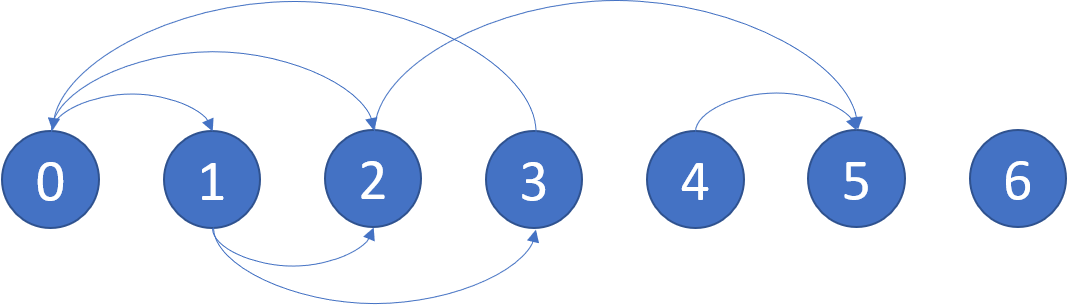# 题目描述：graph 节点数不超过 10000.

# 思路：

1、深度优先遍历每个节点的路径

2、给每个节点设定三种状态，未访问，安全，不安全

3、遍历未访问的节点时，先将节点设置为不安全，若节点的路径中存在不安全的节点，则直接返回该节点是不安全的，若最终没有路径中没有访问到不安全的节点，则返回该节点是安全的。

# 代码：

class Solution {
public:
vector<int> eventualSafeNodes(vector<vector<int>>& graph) {
int len=graph.size();
vector<int>status(len,0);
vector<int>res;
for(int i=0;i<len;i++)
{
if(status[i]==0)function(graph,status,i);
if(status[i]==1)res.push_back(i);
}
return res;
}
bool function(vector<vector<int>>& graph,vector<int>&status,int loc)
{
if(status[loc]==-1)return false;
if(status[loc]==1)return true;
if(graph[loc].size()==0)
{
status[loc]=1;
return true;
}
status[loc]=-1;
for(int i=0;i<graph[loc].size();i++)
{
if(status[graph[loc][i]]==-1)return false;
if(status[graph[loc][i]]==0)
if(!function(graph,status,graph[loc][i]))
return false;
}
status[loc]=1;
return true;
}
};

# 执行效率：

### 内存消耗：46.2 MB, 在所有 C++ 提交中击败了61.57%的用户

06-306608-101万+
04-076206
11-018417
11-295733
07-0724
09-10282
09-291万+
01-27148
04-117149